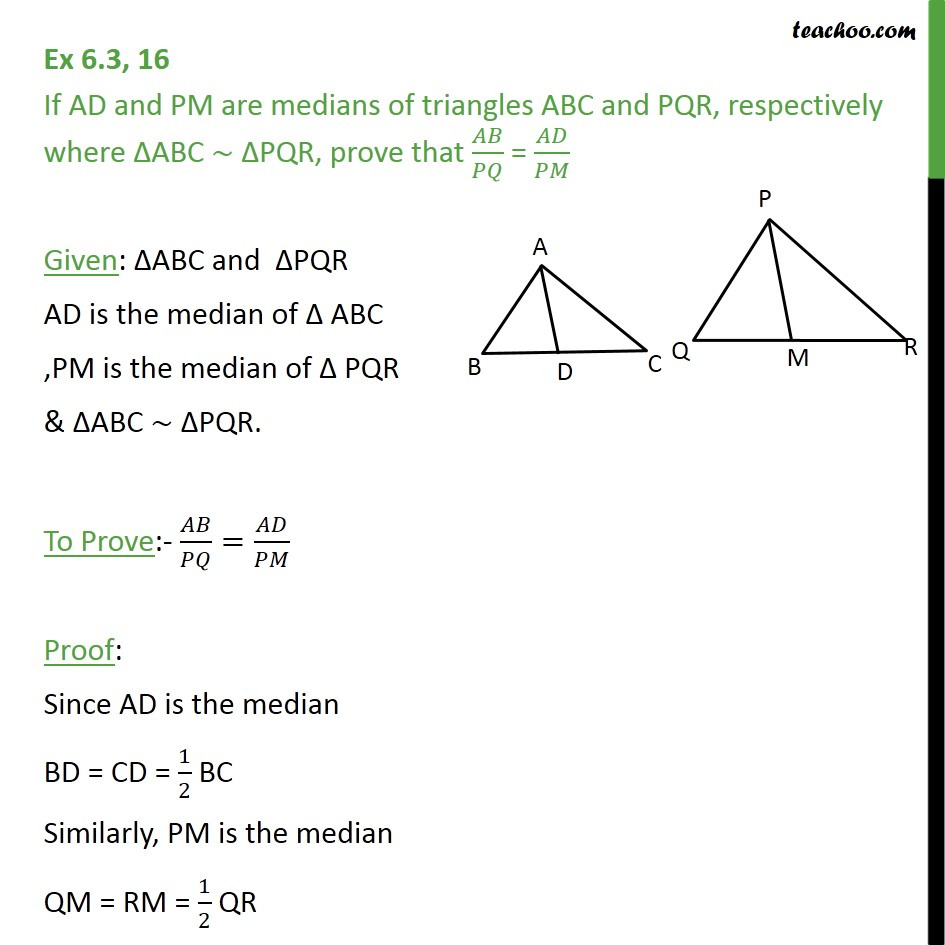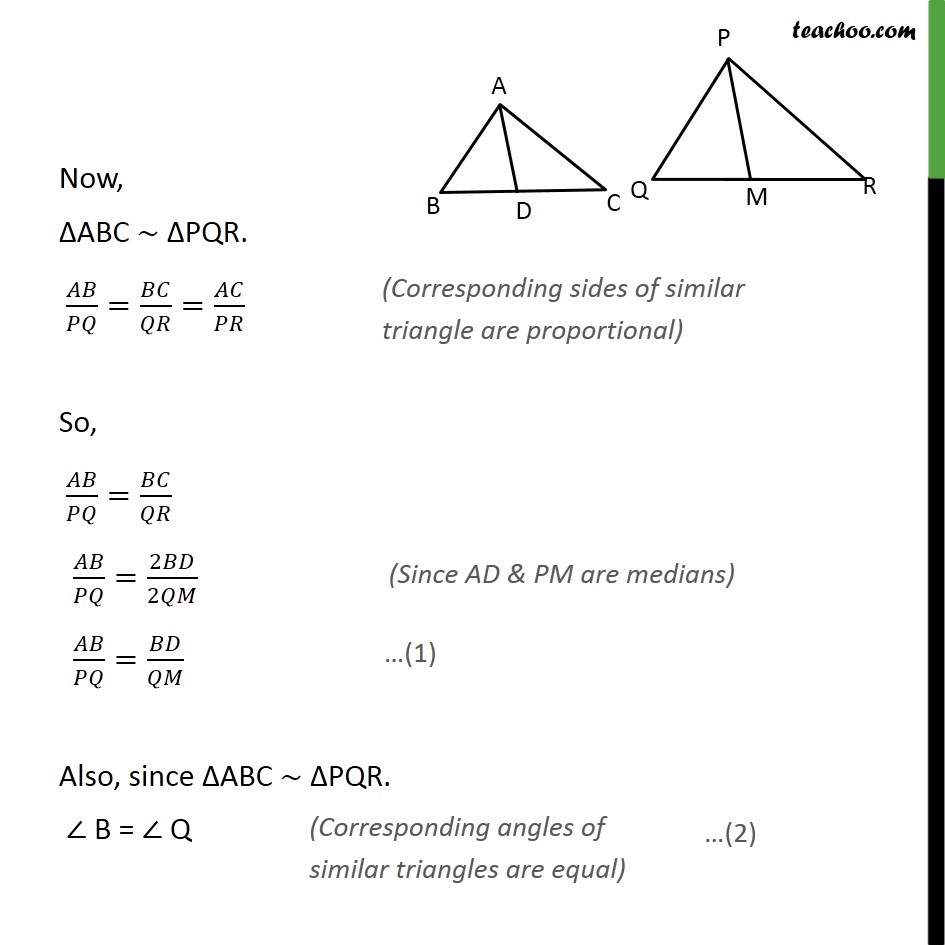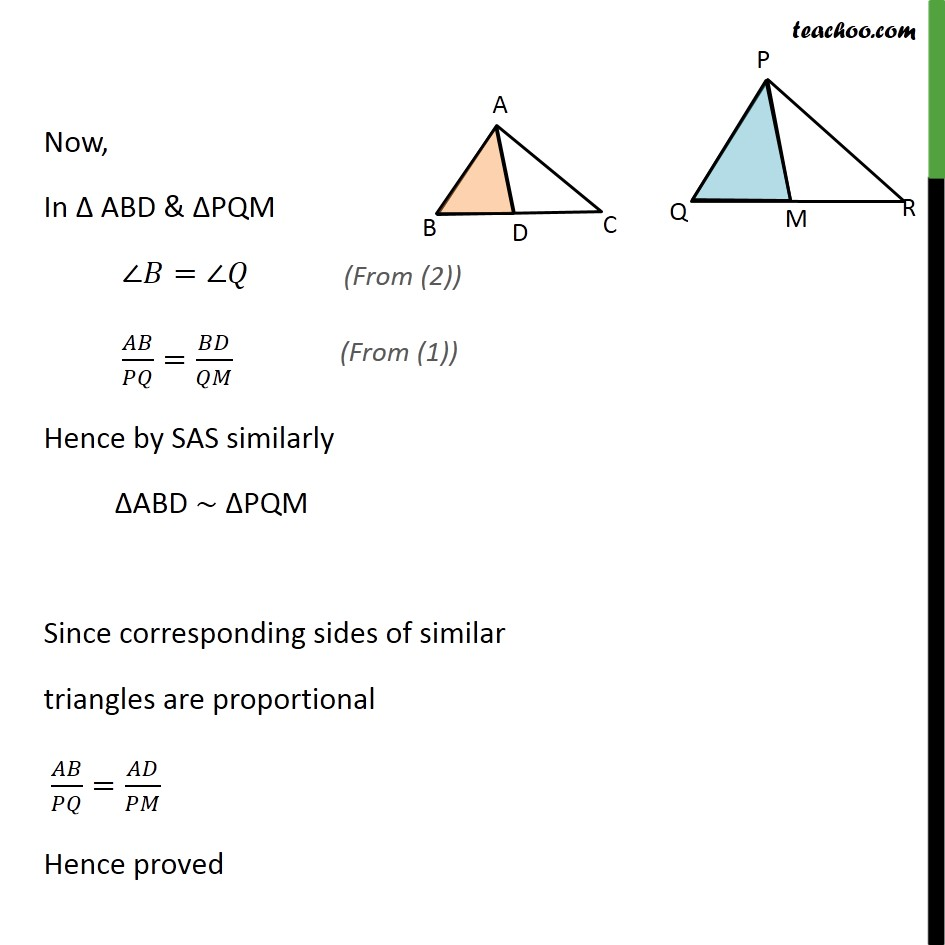1. Chapter 6 Class 10 Triangles
2. Concept wise
3. Given similar, find angles or sides

Transcript

Ex 6.3, 16 If AD and PM are medians of triangles ABC and PQR, respectively where ABC PQR, prove that / = / Given: ABC and PQR AD is the median of ABC ,PM is the median of PQR & ABC PQR. To Prove:- / = / Proof: Since AD is the median BD = CD = 1/2 BC Similarly, PM is the median QM = RM = 1/2 QR Now, ABC PQR. / = / = / So, / = / / =2 /2 / = / Also, since ABC PQR. B = Q Now, In ABD & PQM = / = / Hence by SAS similarly ABD PQM Since corresponding sides of similar triangles are proportional / = / Hence proved

Given similar, find angles or sides